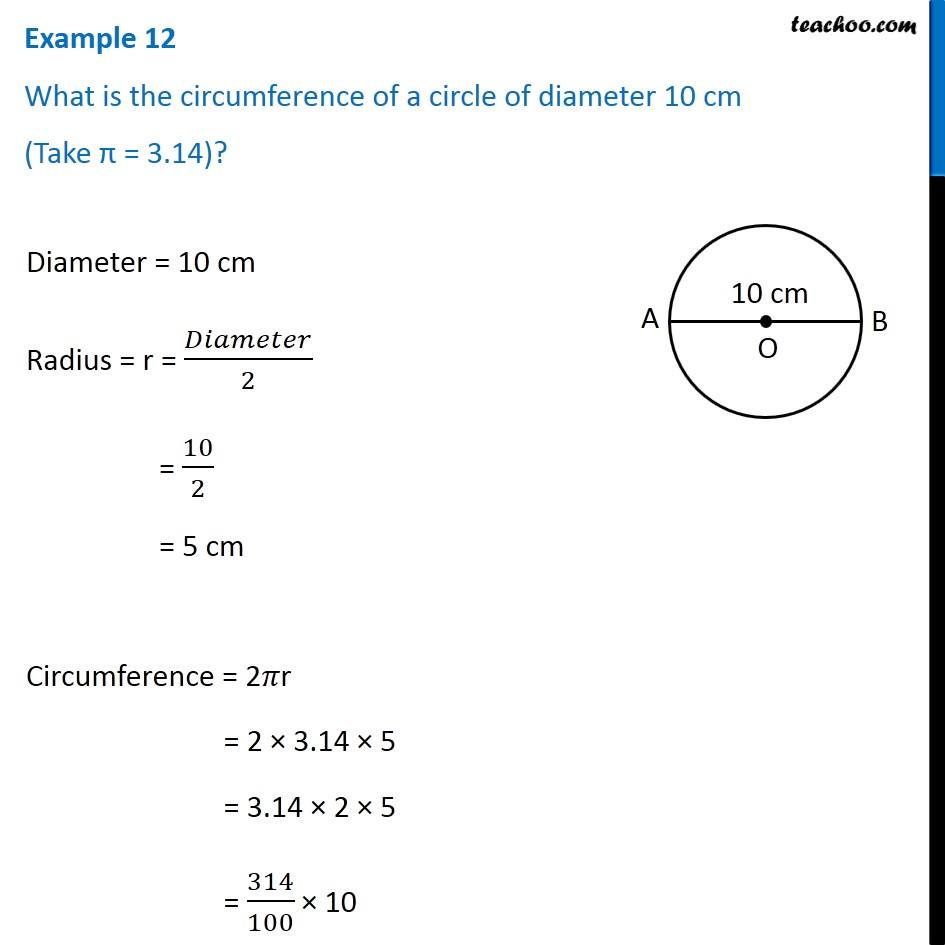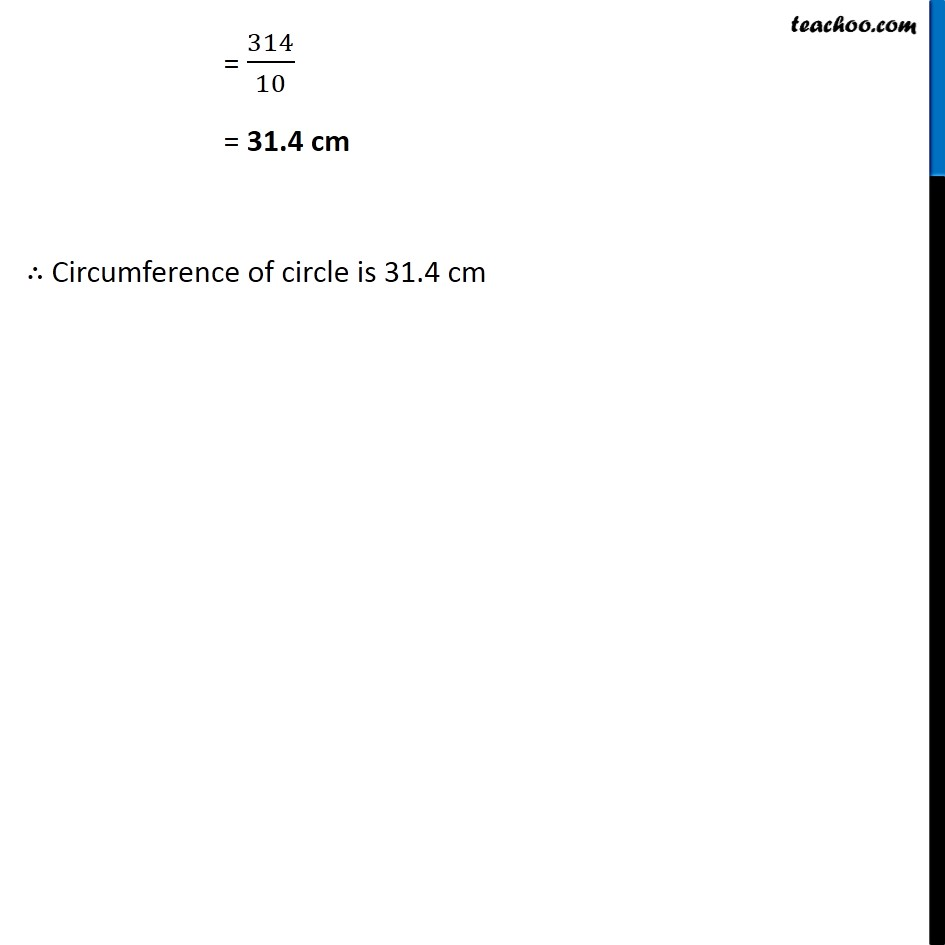1. Chapter 11 Class 7 Perimeter and Area
2. Concept wise
3. Circumference of circle

Transcript

Example 12 What is the circumference of a circle of diameter 10 cm (Take π = 3.14)? Diameter = 10 cm Radius = r = 𝐷𝑖𝑎𝑚𝑒𝑡𝑒𝑟/2 = 10/2 = 5 cm Circumference = 2𝜋r = 2 × 3.14 × 5 = 3.14 × 2 × 5 = 314/100 × 10 = 314/10 = 31.4 cm ∴ Circumference of circle is 31.4 cm